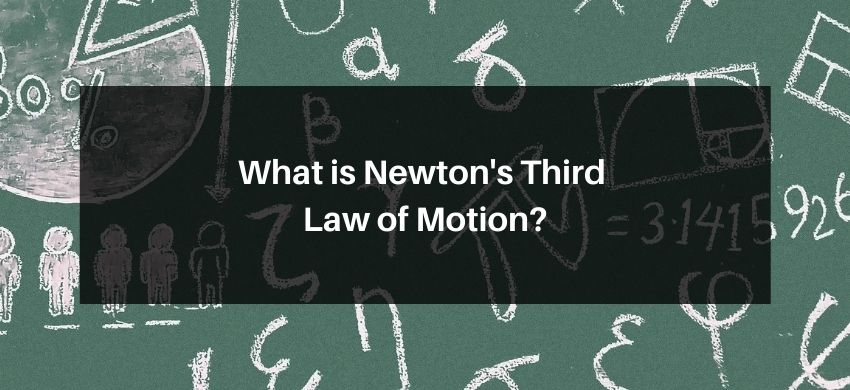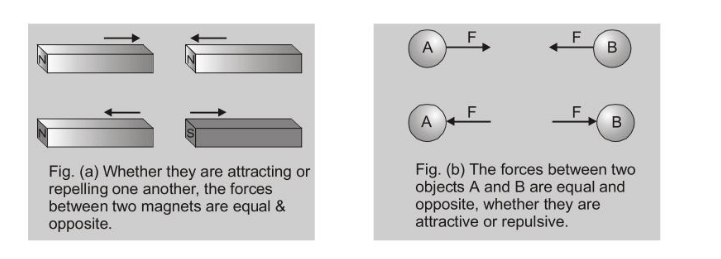Most Affordable JEE | NEET | 8,9,10 Preparation by Kota's Top IITian Doctor Faculties

# What is Newton's third Law of Motion?## Newton's third Law of Motion

To every action there is always equal and opposite reaction or the mutual actions of two bodies upon each other are always directed to contrary parts. The first and second laws are statements about a single object, whereas the third law is a statement about two objects.

[A] According to this law, every action has equal and opposite reaction. Action and reaction act on different bodies and they are simultaneous. There can be no reaction without action.[B] If an object $A$ exerts a force $F$ on an object $B$, then $B$ exerts an equal and opposite force $(-F)$ on $A$.

[C] Newton's III law contradicts theory of relativity, because it states that force signals can travel with infinite speed while theory of relativity states that nothing can travel with a velocity greater than velocity of light.

[D] Action and reaction never cancel each other.

[E] Newton's III law can be derived from II law.

If two particles of masses $m_{1}$ and $m_{2}$ are moving under action of their mutually interacting forces with each other, such that no external force acts on the system.

Let force on $1^{\text {st }}$ due to $2^{\text {nd }}$ is $\vec{F}_{12}=\frac{\overrightarrow{d p}_{1}}{d t}$ (1)

and force on $2^{\text {nd }}$ due to $1^{\text {st }}$ is $\vec{F}_{21}=\frac{\overrightarrow{d p}_{2}}{d t}$ (2)

Adding the two equations, we have $\vec{F}_{12}+\vec{F}_{21}=\frac{\overrightarrow{d p}_{1}}{d t}+\frac{\overrightarrow{d p}_{2}}{d t}=\frac{d}{d t}\left(\vec{p}_{1}+\vec{p}_{2}\right)$

Since no external force acts on the system, the total of momentum of the system must be constant i.e. time rate of change of momentum of the system should be zero. Therefore, the above equation becomes

$\vec{F}_{12}+\vec{F}_{21}=0 \quad$ or $\quad \vec{F}_{21}=-\vec{F}_{12}$